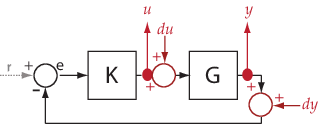# getCompSensitivity

Complementary sensitivity function from generalized model of control system

## Syntax

``T = getCompSensitivity(CL,location)``
``T = getCompSensitivity(CL,location,opening)``

## Description

example

````T = getCompSensitivity(CL,location)` returns the complementary sensitivity measured at the specified location for a generalized model of a control system.```

example

````T = getCompSensitivity(CL,location,opening)` specifies additional loop openings for the complementary sensitivity function calculation. Use an opening, for example, to calculate the complementary sensitivity function of an inner loop, with the outer loop open.If `opening` and `location` list the same point, the software opens the loop after adding the disturbance signal at the point.```

## Examples

collapse all

Compute the complementary sensitivity at the plant output, `X`, of the control system of the following illustration.Create a model of the system by specifying and connecting a numeric LTI plant model `G`, a tunable controller `C`, and the `AnalysisPoint` block `X`. Use the `AnalysisPoint` block to mark the location where you assess the complementary sensitivity, which in this example is the plant output.

```G = tf(,[1 5]); C = tunablePID('C','p'); C.Kp.Value = 3; X = AnalysisPoint('X'); CL = feedback(G*C,X);```

`CL` is a `genss` model that represents the closed-loop response of the control system from r to y. Examine the Control Design Blocks of the model.

`CL.Blocks`
```ans = struct with fields: C: [1x1 tunablePID] X: [1x1 AnalysisPoint] ```

The model's blocks include the `AnalysisPoint` block, `X`, that identifies the analysis-point location.

Calculate the complementary sensitivity, T, at `X`.

`T = getCompSensitivity(CL,'X')`
```T = Generalized continuous-time state-space model with 1 outputs, 1 inputs, 1 states, and the following blocks: C: Tunable PID controller, 1 occurrences. X: Analysis point, 1 channels, 1 occurrences. Type "ss(T)" to see the current value, "get(T)" to see all properties, and "T.Blocks" to interact with the blocks. ```

`getCompSensitivity` preserves the Control Design Blocks of `CL`, and returns a `genss` model. To get a numeric model, you can convert `T` to transfer-function form, using the current value of the tunable block.

`Tnum = tf(T)`
```Tnum = From input "X" to output "X": -3 ----- s + 8 Continuous-time transfer function. ```

In the multiloop system of the following illustration, calculate the inner-loop sensitivity at the output of `G2`, with the outer loop open.Create a model of the system by specifying and connecting the numeric plant models, tunable controllers, and `AnalysisPoint` blocks. `G1` and `G2` are plant models, `C1` and `C2` are tunable controllers, and `X1` and `X2` are `AnalysisPoint` blocks that mark potential loop-opening locations.

```G1 = tf(10,[1 10]); G2 = tf([1 2],[1 0.2 10]); C1 = tunablePID('C','pi'); C2 = tunableGain('G',1); X1 = AnalysisPoint('X1'); X2 = AnalysisPoint('X2'); CL = feedback(G1*feedback(G2*C2,X2)*C1,X1);```

Calculate the complementary sensitivity, $T$, at `X2`, with the outer loop open at `X1`. Specifying `X1` as the third input argument tells `getCompSensitivity` to open the loop at that location.

```T = getCompSensitivity(CL,'X2','X1'); tf(T)```
```ans = From input "X2" to output "X2": -s - 2 ---------------- s^2 + 1.2 s + 12 Continuous-time transfer function. ```

## Input Arguments

collapse all

Model of a control system, specified as a generalized state-space model (`genss`).

Locations at which you can perform sensitivity analysis or open loops are marked by `AnalysisPoint` blocks in `CL`. Use `getPoints(CL)` to get the list of such locations.

Location at which you calculate the complementary sensitivity function, specified as a character vector or cell array of character vectors. To extract the complementary sensitivity function at multiple locations, use a cell array of character vectors.

Each specified location must match an analysis point in `CL`. Analysis points are marked using `AnalysisPoint` blocks. To get the list of available analysis points in `CL`, use `getPoints(CL)`.

Example: `'u'` or `{'u','y'}`

Additional loop opening used to calculate the complementary sensitivity function, specified as a character vector or cell array of character vectors. To open the loop at multiple locations, use a cell array of character vectors.

Each specified opening must match an analysis point in `CL`. Analysis points are marked using `AnalysisPoint` blocks. To get the list of available analysis points in `CL`, use `getPoints(CL)`.

Use an opening, for example, to calculate the complementary sensitivity function of an inner loop, with the outer loop open.

If `opening` and `location` list the same point, the software opens the loop after adding the disturbance signal at the point.

Example: `'y_outer'` or `{'y_outer','y_outer2'}`

## Output Arguments

collapse all

Complementary sensitivity function of the control system, `T`, measured at `location`, returned as a generalized state-space model (`genss`).

• If `location` specifies a single analysis point, then `T` is a SISO `genss` model.

• If `location` is a vector signal, or specifies multiple analysis points, then `T` is a MIMO `genss` model.

collapse all

### Complementary Sensitivity

The complementary sensitivity function, T, at a point is the closed-loop transfer function around the feedback loop measured at the specified location. It is related to the open-loop transfer function, L, and the sensitivity function, S, at the same point as follows:

`$T=\frac{L}{1-L}=S-1.$`

Use `getLoopTransfer` and `getSensitivity` to compute L and S.

Consider the following model:The complementary sensitivity, T, at `y` is defined as the transfer function from `dy` to `y`.Observe that, in contrast to the sensitivity function, the disturbance, `dy`, is added after the measurement, `y`.

`$\begin{array}{l}y=-GK\left(y+dy\right)\\ \to y=-GKy-GKdy\\ \to \left(I+GK\right)y=-GKdy\\ \to y=\underset{T}{\underbrace{-{\left(I+GK\right)}^{-1}GK}}dy.\end{array}$`

Here, I is an identity matrix of the same size as GK. The complementary sensitivity transfer function at `y` is equal to `-1` times the closed-loop transfer function from `r` to `y`.

Complementary sensitivity at multiple locations, for example, `u` and `y`, is defined as the MIMO transfer function from the disturbances to measurements:`$T=\left[\begin{array}{cc}{T}_{du\to u}& {T}_{dy\to u}\\ {T}_{du\to y}& {T}_{dy\to y}\end{array}\right].$`

## Version History

Introduced in R2014a